# Question 7 The data below refer to the following reaction: 2AB (g) Az (g)+ B2(g) and...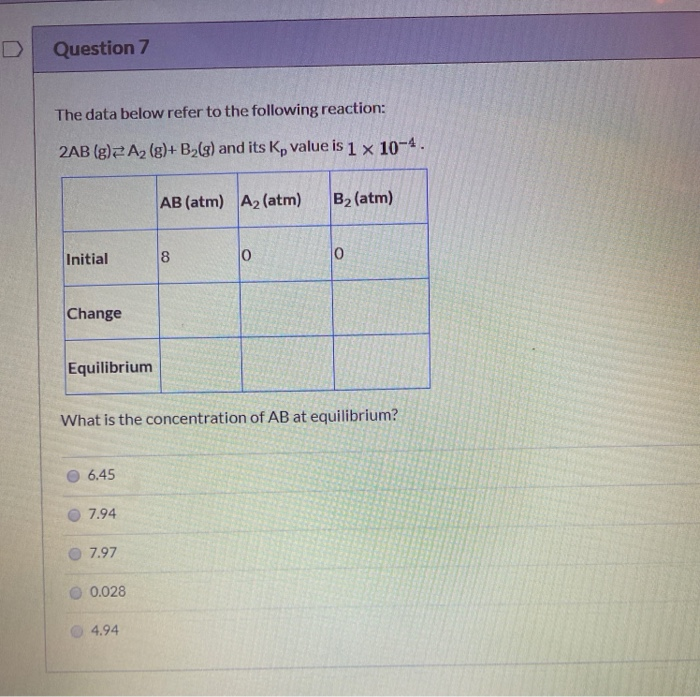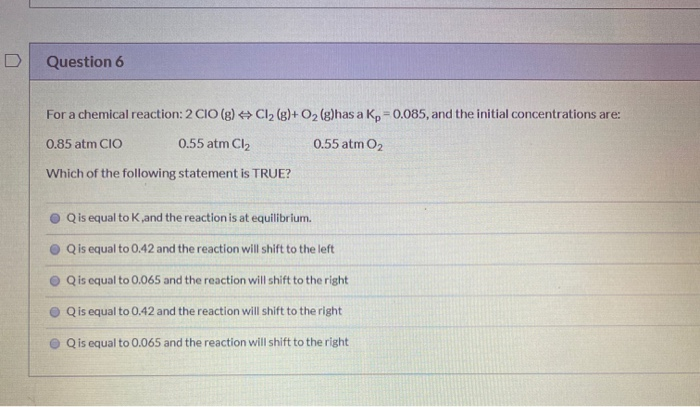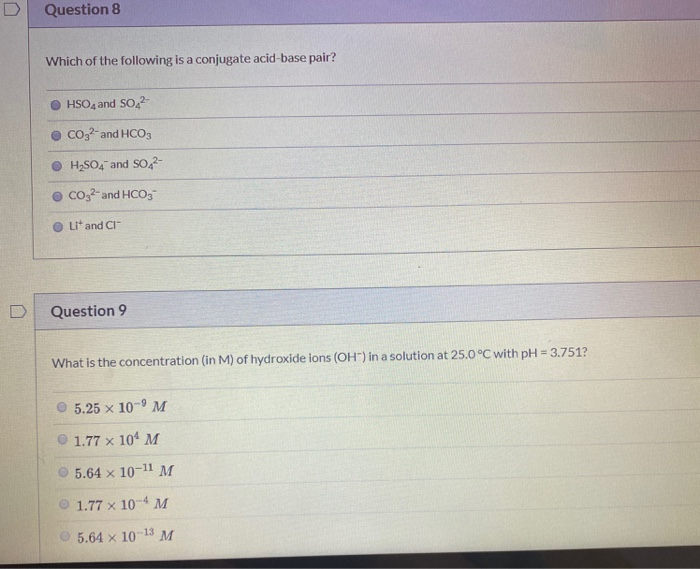Question 7 The data below refer to the following reaction: 2AB (g) Az (g)+ B2(g) and its Kp value is 1 x 10-4. AB (atm) A2 (atm) B2 (atm) Initial 8 0 0 Change Equilibrium What is the concentration of AB at equilibrium? 6.45 7.94 7.97 0.028 4.94
Question 6 For a chemical reaction: 2 CIO(g) + Cl2(g)+ O2(g)has a Kp = 0.085, and the initial concentrations are: 0.85 atm CIO 0.55 atm C12 0.55 atm O2 Which of the following statement is TRUE? Qis equal to K and the reaction is at equilibrium. Qis equal to 0.42 and the reaction will shift to the left Q is equal to 0.065 and the reaction will shift to the right Q is equal to 0.42 and the reaction will shift to the right Q is equal to 0.065 and the reaction will shift to the right
Question 8 Which of the following is a conjugate acid-base pair? HSO4 and SO2 CO32- and HCO3 H2SO4 and SO2- CO32- and HCO3 Lit and CI Question 9 What is the concentration (in M) of hydroxide ions (OH') in a solution at 25.0°C with pH = 3.751? 5.25 x 10-9 M 1.77 x 104 M 5.64 x 10-11 M 1.77 x 10-4 M 5.64 x 10-13 M

Here is the Solution: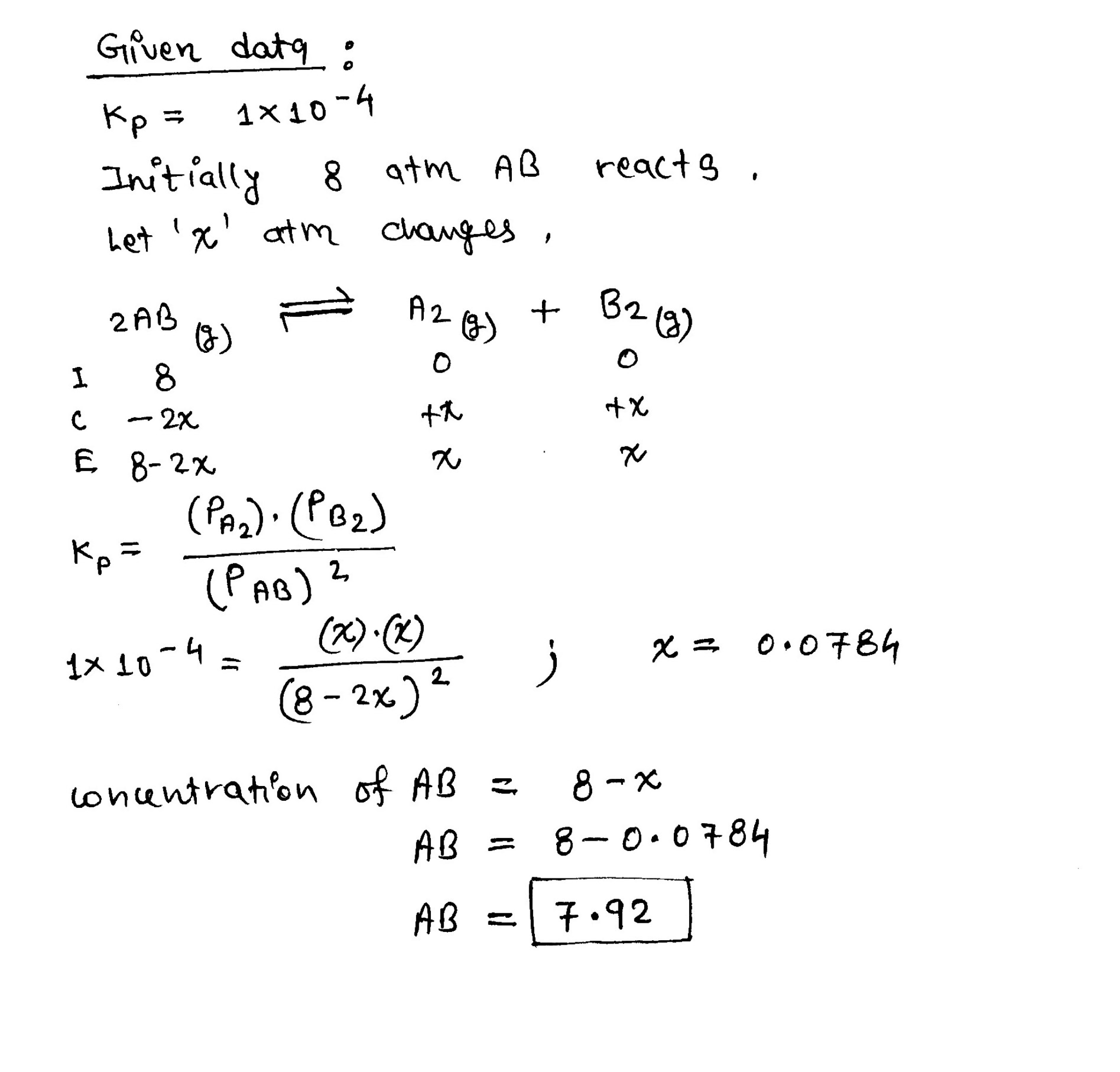Thanks! :-)

##### Add Answer of: Question 7 The data below refer to the following reaction: 2AB (g) Az (g)+ B2(g) and...
Similar Homework Help Questions
• ### The equilibrium constant, Kp, for the following reaction is 2.74 at 1.15x103K. 2803(g) 22502(g) + O2(g)...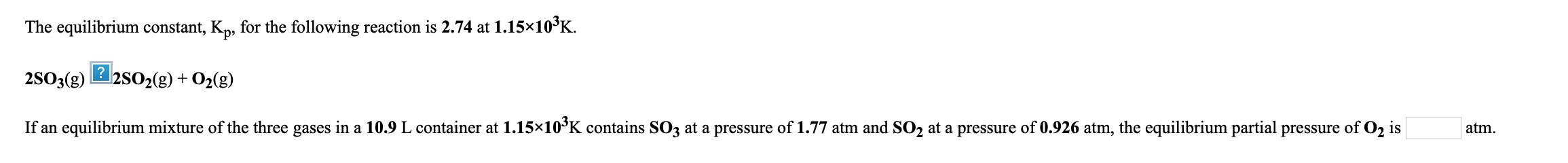The equilibrium constant, Kp, for the following reaction is 2.74 at 1.15x103K. 2803(g) 22502(g) + O2(g) + If an equilibrium mixture of the three gases in a 10.9 L container at 1.15*10²K contains SO3 at a pressure of 1.77 atm and SO2 at a pressure of 0.926 atm, the equilibrium partial pressure of O2 is atm. Show transcribed image text

• ### At 400K, the equilibrium constant for the reaction Br2(g) + Cl2(g) ⇌ 2BrCl(g) is KP =...

At 400K, the equilibrium constant for the reaction Br2(g) + Cl2(g) ⇌ 2BrCl(g) is KP = 7.0. A closed vessel at 400K is charged with 1.00 atm of Br2(g), 1.00 atm of Cl2(g), and 2.00 atm of BrCl(g). Use Q to determine which of the statements below is true. A. The equilibrium partial pressure of BrCl(g) will be less than 2.00 atm B. The reaction will go to completion since there are equal amounts of Br2 and Cl2 C. At...

• ### QUESTION 10 [CLO-6] At 900 K, the equilibrium constant (Kp) for the following reaction is 0.345....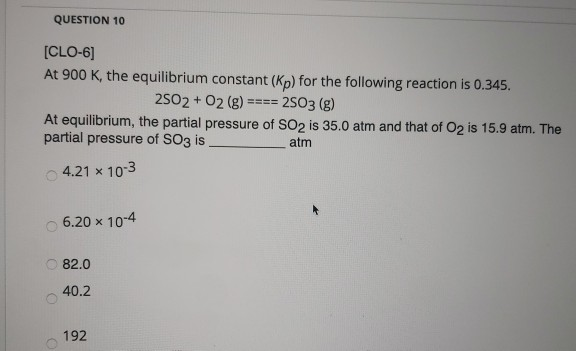QUESTION 10 [CLO-6] At 900 K, the equilibrium constant (Kp) for the following reaction is 0.345. 2502 + O2(g) ==== 2503 (g) At equilibrium, the partial pressure of SO2 is 35.0 atm and that of O2 is 15.9 atm. The partial pressure of SO3 is atm 4.21 x 10-3 6.20 x 10-4 82.0 40.2 192

• ### Consider the following reaction, 2AB (g) ⇌ A2 (g) + B2 (g) K = 1.50x10−2 At...

Consider the following reaction, 2AB (g) ⇌ A2 (g) + B2 (g) K = 1.50x10−2 At some point in the reaction, Q = 5.30. Which of the following statements is correct concerning the equilibrium? 0.60 mol of Br2 and 0.60 mol of Cl2 are placed in a 1.00 L flask and allowed to reach equilibrium. After reaching equilibrium, the flask is found to contain 0.28 mol of BrCl. What is the value of Kc for this reaction? Br2  (g) + Cl2  (g)...

• ### The following reaction: 2SO3 (g)

The following reaction: 2SO3 (g) ! 2SO2 (g) + O2 (g) has an equilibrium constant equal to 0.23 M. If the following concentrations are present: [SO2] =0.480 M, [O2] = 0.561 M, [SO3] = 0.220 M, is the reaction at equilibrium? If not, which way must it shift to reach equilibrium?

• ### Be sure to answer all parts. At 430°C, the equilibrium constant (Kp) for the reaction 2NO(g)+O2()s 2NO2(g) is 1.5 x...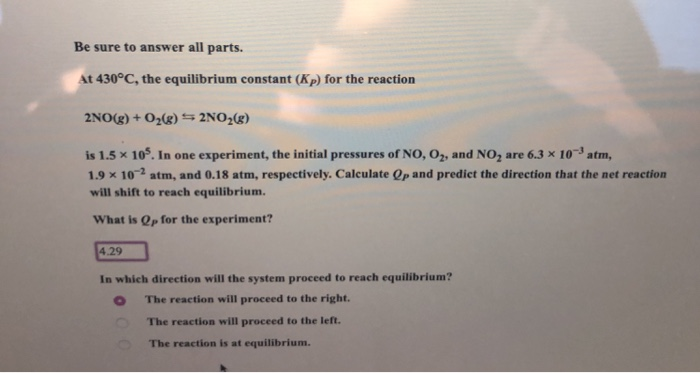Be sure to answer all parts. At 430°C, the equilibrium constant (Kp) for the reaction 2NO(g)+O2()s 2NO2(g) is 1.5 x 105. In one experiment, the initial pressures of NO, O2, and NO are 6.3 x 103 atm, 1.9 x 10-2 atm, and 0.18 atm, respectively. Calculate Op and predict the direction that the net reaction will shift to reach equilibrium. What is Qp for the experiment? 4.29 In which direction will the system proceed to reach equilibrium? The reaction will...

• ### The equilibrium constant is equal to 5.00 at 1300 K for the reaction: 2SO2(g) + O2(g)...

The equilibrium constant is equal to 5.00 at 1300 K for the reaction: 2SO2(g) + O2(g) ⇌ 2SO3(g) If initial concentrations are [SO2] = 10.8 M, [O2] = 0.45 M, and [SO3] = 16.2 M, the system is? Group of answer choices not at equilibrium and will shift to the left to achieve an equilibrium state. not at equilibrium and will shift to the right to achieve an equilibrium state. not at equilibrium and will remain in an unequilibrated state....

• ### The equilibrium constant is equal to 5.00 at 1300 K for the reaction: 2502(g) + O2(g)...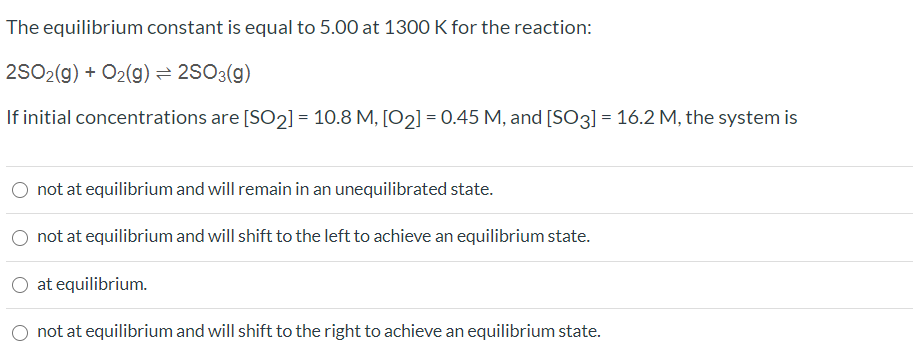The equilibrium constant is equal to 5.00 at 1300 K for the reaction: 2502(g) + O2(g) = 2503(g) If initial concentrations are [SO2] = 10.8 M, [O2] = 0.45 M, and (SO3) = 16.2 M, the system is not at equilibrium and will remain in an unequilibrated state. not at equilibrium and will shift to the left to achieve an equilibrium state. O at equilibrium. not at equilibrium and will shift to the right to achieve an equilibrium state. Show...

• ### Which of the following expressions is the correct equilibrium-constant expression for the reaction below? 200(g) =...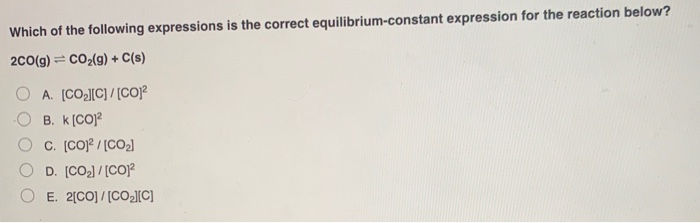Which of the following expressions is the correct equilibrium-constant expression for the reaction below? 200(g) = CO2(g) + C(s) A. [CO][C]/[CO] B. K [CO] C. [CO] / [CO2) D. [CO]/[CO] E. 2[CO] /[CO][C] In which of the following reactions would increasing pressure at constant temperature not change the concentrations of reactants and products, based on Le Châtelier's principle? ООООО A. 2N, () + 0,02N,0 () B. N.O. (g) 2NO, (g) C. N2(g) + 3H2(g)2NH3 (9) D. N2 (9) + 202...

• ### At 745K, the reaction 2AB(g) ? A2(g) + B2(g) has a Kc = 2.00 x 10–2. If a mixture of 1.00 mol...

At 745K, the reaction 2AB(g) ? A2(g) + B2(g) has a Kc = 2.00 x 10–2. If a mixture of 1.00 mol AB and 1.00 mol A2 is sealed into a 10.0 L flask and heated to 745K, whatwill be the equilibrium concentration of B2?

Free Homework App